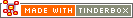max(set)Operator Type: Set maths Operator Scope of Action: Set

The max() operator returns the largest item in a set.

Both the operators max() and min() use lexical comparison in most cases, but numeric comparison if the context is numeric, e.g.:

`Width=max("1;100;2")`

Since "Width" is numeric, max() will be return 100.

`Name=max("1;100;2")`

Since "Name" is a string, max() will return 2.

If you don't have a set, create one on the fly:

`MyMax = max(collect(all,\$MyNum))`

A 'lexical sort' is a case-insensitive sort (Aardvark,aarvark,ABLE) and for numbers "2;70;100;" would sort in order "100;2;70" (i.e. ordered on first number first - a number 'computer sort' )

 Up: Full Operator List Previous: lowercase(data) Next: min(set)

[Last updated: 14 Dec 2009, using v5.0]